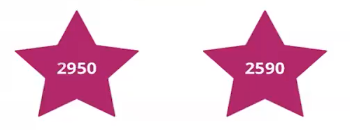Study P3 Mathematics Whole Numbers 1 - Geniebook# Whole Numbers 1

Whole numbers are the basic counting numbers 0, 1, 2, 3, 4, 5, 6 and so on. In P3, we learn numbers up to 10 000.

1. Reading and writing numbers up to 10 000
2. Comparing and ordering numbers

## 1. Reading And Writing Numbers Up To 10 000

When writing numbers more than 1000 in words, we need to add a comma to separate the hundreds from the thousands.

Question 1:

Write the following in words.

1508

One thousand, five hundred and eight

Question 2:

Write the following in words.

3520

Three thousand, five hundred and twenty

Question 3:

Write the following in words.

7845

Seven thousand, eight hundred and forty-five

Question 4:

Write the following in words.

10 000

Ten thousand

Question 5:

Write the following in numerals.

Six thousand, five hundred and nineteen

1. 6519
2. 6590
3. 6591
4. 5619

(1) 6519

Question 6:

Write the following in numerals.

Two thousand and seventy-five

1. 2750
2. 2705
3. 2275
4. 2075

(4) 2075

Question 7:

Write the following in numerals.

Four thousand and nine

1. 409
2. 4009
3. 4090
4. 4900

(2) 4009

Question 8:

Write the following in numerals.

Five thousand, four hundred and eleven

5411

Question 9:

Write the following in numerals.

Nine thousand, two hundred and five

9205

Question 10:

Which of the following has the same value as the words written below?

Nine thousand, one hundred and thirteen

1. 9000 $$+$$ 10 $$+$$ 13
2. 9000 $$+$$ 100 $$+$$ 13
3. 9000 $$+$$ 10 $$+$$ 30
4. 9000 $$+$$ 100 $$+$$ 130

Solution:

9000 $$+$$ 100 $$+$$ 13 $$=$$ 9113

(2) 9000 $$+$$ 100 $$+$$ 13

## 2. Comparing And Ordering Numbers

When we compare numbers, we will compare the digits from the left to the right.

The greater the digit on the “left”, the greater the number.

Question 1:

Let’s take a look at the following numbers:Which number is greater?

Solution:

The digit in the thousands place of both numbers is 2.
Since they are the same, we look at the digit in the hundreds place.

The digit in the hundreds place of both numbers is different. We compare them.
Since 9 is greater than 5, 2950 is greater than 2590.

2950

Question 2:

Which of the following is the greatest?

1. 6710
2. 2375
3. 4097
4. 1684

Solution:

By comparing the digits in the thousands place for all the numbers, 6 is the greatest.
Hence, 6710 is the greatest number.

(1) 6710

Question 3:

Which of the following is the smallest?

1. 7835
2. 1089
3. 2550
4. 4872

Solution:

By comparing the digits in the thousands place for all the numbers, 1 is the smallest.
Hence, 1089 is the smallest number.

(2) 1089

Question 4:

Which of the following is the greatest?

1. 5760
2. 5670
3. 6750
4. 6570

Solution:

By comparing the digits in the thousands place for all the numbers, 6 is greater than 5.
Hence, we compare 6750 and 6570.

By comparing the digits in the hundreds place for 6750 and 6570, 7 is greater than 5.
Hence, 6750 is the greatest number.

(3) 6750

Question 5:

Which of the following is the smallest?

1. 2323
2. 2332
3. 3232
4. 3223

Solution:

By comparing the digits in the thousands place for all the numbers, 2 is smaller than 3.
Hence, we compare 2323 and 2332.

The digit in the hundreds place for 2323 and 2332 is the same. By comparing the digits in the tens place for 2323 and 2332, 2 is smaller than 3.
Hence, 2323 is the smallest number.

(1) 2323

Question 6:

Arrange the following numbers in order, starting with the smallest.

 3750 573 3705 5370

Solution:

 573 , 3705 , 3750 , 5370 smallest

Question 7:

Arrange the following numbers in order, starting with the greatest.

 4554 454 4455 5445

Solution:

 5445 , 4554 , 4455 , 454 greatest

Question 8:

Arrange the following numbers in order, starting with the smallest.

 1095 5091 9051 1509

Solution:

 1095 , 1509 , 5091 , 9051 smallest

Question 9:

Arrange the following numbers in order, starting with the greatest.

 3500 5030 3050 5300

Solution:

 5300 , 5030 , 3500 , 3050 greatest

Question 10:

Arrange the following numbers in order, starting with the smallest.

 2960 2680 2806 2608

Solution:

 2608 , 2680 , 2806 , 2960 smallest

Question 11:

Arrange the following numbers in order, starting with the greatest.

 1001 1100 1011 1010

Solution:

 1100 , 1011 , 1010 , 1001 greatest

Continue Learning
Volume Money
Mass Fractions
Addition And Subtraction Whole Numbers 1
Whole Numbers 3 Whole Numbers 6
Bar Graphs Angles
Multiplication and Division Length, Mass And Volume
Length Area and Perimeter
Parallel And Perpendicular LinesPrimaryPrimary 1Primary 2Primary 3EnglishMaths
Volume
Money
Mass
Fractions
Whole Numbers 1
Whole Numbers 3
Whole Numbers 6
Bar Graphs
Angles
Multiplication and Division
Length, Mass And Volume
Length
Area and Perimeter
Parallel And Perpendicular LinesSciencePrimary 4Primary 5Primary 6SecondarySecondary 1Secondary 2Secondary 3Secondary 4